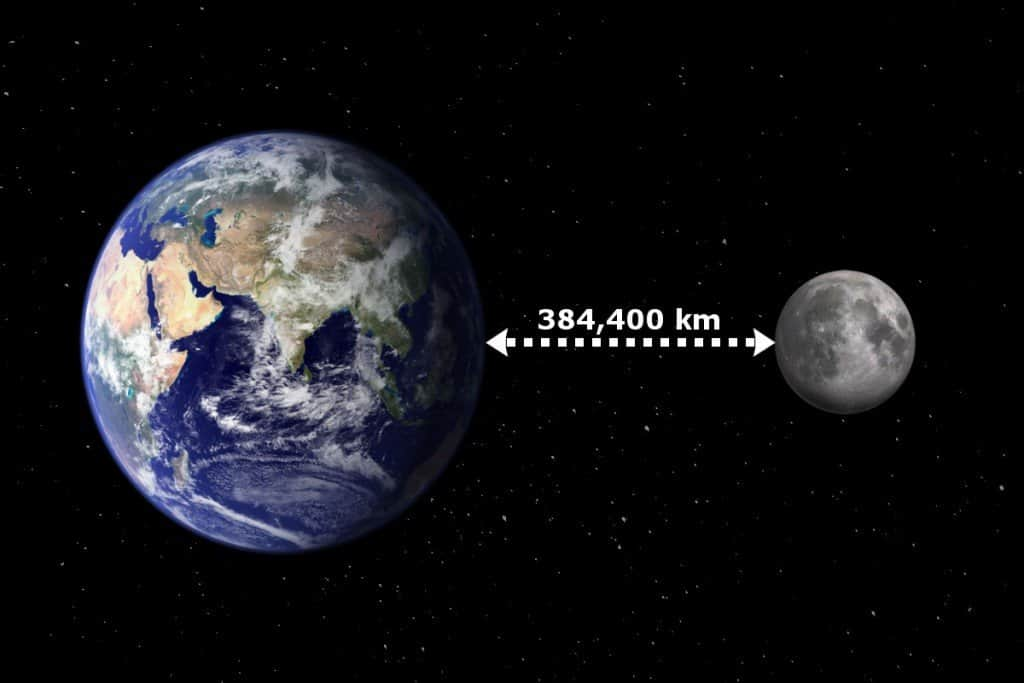# How Far Is the Moon From Earth?How Far Is the Moon From Earth?

Introduction:
The Moon, Earth’s only natural satellite, has fascinated humans for centuries. Its proximity to our planet has allowed us to study and explore its surface, leading to numerous discoveries and advancements in our understanding of the universe. One question that often arises is: how far is the Moon from Earth? In this article, we will delve into the intricacies of this celestial distance, exploring the various measurements and methods used to determine the Moon’s exact location in space.

1. Average Distance:
When discussing the distance between the Moon and Earth, scientists commonly refer to the average distance. This value is calculated by taking the mean of the Moon’s closest point (perigee) and its farthest point (apogee) from our planet. The average distance between the Moon and Earth is approximately 238,855 miles (384,400 kilometers). However, it is important to note that this distance can vary due to the Moon’s elliptical orbit around Earth.

The Moon’s elliptical orbit means that its distance from Earth is not constant throughout its revolution. At perigee, when the Moon is closest to Earth, it can be as near as 225,623 miles (363,104 kilometers). Conversely, at apogee, when it is farthest from Earth, the Moon can be as distant as 252,088 miles (405,696 kilometers). These variations in distance have significant implications for phenomena such as tides on Earth.

2. Measuring Techniques:
Determining the precise distance between the Moon and Earth requires sophisticated measurement techniques. One such method is called lunar laser ranging. It involves firing a laser beam at retroreflectors placed on the Moon’s surface during various Apollo missions. By measuring the time it takes for the laser beam to return to Earth, scientists can calculate the distance with remarkable accuracy.

Another technique used to measure the Moon’s distance is radar ranging. By bouncing radio waves off the Moon’s surface and timing their return, scientists can calculate the time it takes for the waves to travel to the Moon and back. This information allows them to determine the distance between Earth and the Moon.

3. Historical Measurements:
Throughout history, scientists and astronomers have made significant efforts to estimate the distance between the Moon and Earth. One of the earliest attempts was made by the ancient Greek astronomer Hipparchus in the second century BCE. Using trigonometry, he calculated the Moon’s distance based on observations of lunar eclipses. Although his estimation was not entirely accurate, it laid the foundation for future calculations.

In the 17th century, Johannes Kepler proposed a method to determine the Moon’s distance by comparing its apparent size to that of the Sun during a solar eclipse. This method, known as parallax, involves measuring the angular difference between two points of observation on Earth. While Kepler’s approach was a step forward, it still lacked the precision required for an accurate measurement.

4. Significance of Knowing the Distance:
Understanding the precise distance between the Moon and Earth is crucial for various scientific endeavors. For instance, it plays a vital role in space exploration missions. NASA and other space agencies use this information to plan trajectories, calculate fuel requirements, and ensure accurate navigation for spacecraft traveling to the Moon.

Moreover, knowing the Moon’s distance allows scientists to study its gravitational influence on Earth. The Moon’s gravitational pull is responsible for phenomena such as ocean tides and subtle changes in Earth’s rotation. Accurate measurements of this distance help scientists model and predict these effects, contributing to our understanding of Earth’s dynamics.

Conclusion:
The Moon’s distance from Earth is approximately 238,855 miles (384,400 kilometers) on average. However, due to its elliptical orbit, this distance can vary between 225,623 miles (363,104 kilometers) at perigee and 252,088 miles (405,696 kilometers) at apogee. Scientists employ advanced techniques like lunar laser ranging and radar ranging to measure this distance accurately. Understanding the Moon’s distance is not only important for scientific research but also for planning space missions and comprehending the Moon’s impact on Earth. As we continue to explore the mysteries of the universe, knowing the exact distance between the Moon and Earth remains a fundamental piece of knowledge.